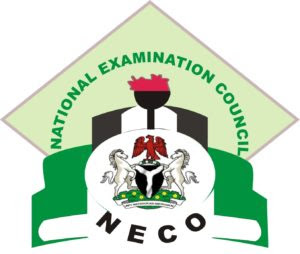## Wednesday, 14 June 2017

### NECO 2017 Mathematics Questions Online [OBJ and THEORY Answers].

Note that this is NECO Mathematics Questions 2017 | The National Examinations Council is an examination body in Nigeria that conducts the Senior Secondary Certificate Examination and the General Certificate in Education in June/July and December/January respectively.Read below NECO 2017 Mathematics Questions Online

1b)
I = PRT/100, p=N15000 R=10% and I=3years
A = P+ I
where I = 15000*10*3/100=N4500
A=4500+15000 =N19500

1b)
I = PRT/100, p=N15000 R=10% and I=3years
A = P+ I
where I = 15000*10*3/100=N4500
A=4500+15000 =N19500

==================================

2a)
using sine rule

b/sin20 = 6/sin30
bsin30 = 6sin120

b 6sin120/sin30
b = 6×0.2511/0.4540
b = 5.7063/0.4540
b = 12.57 ≠ 12.6cm

2bi)
the diagram is euivalent triangles.

where
|AX|/|BC| = |BY|/|AC| = |XY|/|YC|
XY = 9, BY = 7
YC = 18-7=11
9/11 = 7/|AC|
9|AC| = 77
|AC| = 77/9
|AC| = 8cm

2bii)
XY/AB = BY/AC
9/|AB| = 7/8.6
|AB| = 9×8.6/7
|AB| = 11cm